The Demand for Money in Nigeria: Evidence from Bounds Testing Approach | OMICS International

# The Demand for Money in Nigeria: Evidence from Bounds Testing Approach

Martins Iyoboyi1*, Latifa M Pedro2

1Department of Economics and Development Studies, Federal University Dutsin-Ma, Katsina, Nigeria.

2Department of Economics, Faculty of the Social Sciences, Bayero University, Kano, Nigeria.

*Corresponding Author:
Martins Iyoboyi
Department of Economics and Development Studies
Federal University Dutsin-Ma, Katsina, Nigeria
Email: [email protected], [email protected]

Accepted date: April 04, 2013; Published date: April 16, 2013

Visit for more related articles at Business and Economics Journal

#### Abstract

This case study offers an insider’s account of financial industry developments from 1999 to 2007 as emerging evidence of the global contagion of a mind-set and the effects of “reverse legitimacy”, or undue influence of powerful financial firms embracing this mind-set, upon societal institutions. The study seeks to explain why, despite clear indications that housing prices had inflated over the first decade of the millennium, that the expansion of credit had exploded, and that financial models on Wall Street – which are by definition, mathematical simplifications of real-world complexities that assume “normal” market conditions and thus do not factor for speculative bubbles or unexpected shocks to the system – financial and regulatory institutions remained confident that everything was basically under control. Loan data are provided as emerging evidence of conformity in industry behavior. Selected excerpts from letters to shareholders in the 2007 annual reports of six major banks are presented as partial evidence of cognitive impairment in organizational thinking in the years leading up to the banks’ financial collapse in 2008.

#### Keywords

Autoregressive distributed lag; cointegration; demand for money; monetary policy; vector error correction.

#### Introduction

There is a plethora of studies which investigate the determinants of money demand across countries and regions. Consequently, estimating the money demand function attracted many scholars, due in part to the policy implications originating from them are likely to have on the countries or regions studied.

There has been extensive investigation on the demand for money and its determinants in developing countries. The studies include those conducted on African countries by Simmons , Nwafor et al. , Fielding , Nell , Owoye and Onafowora , Kallon , Akinlo , Bahmani-Oskooee and Gelan , Ghartey  and Drama and Yao . Studies on the demand for money on Asian economies include those of Bahmani-Oskooee and Rhee  and Arize . Other studies on developing countries include Halicioglu et al. , Agénor and Khan  and Valdkhani and Alauddin . Thus, among economists interest has been intense in terms of correctly estimating and forecasting the efficacy of monetary policy in developing countries .

The monetary authorities have shown increasing appetite for stable money demand as a pivotal for conducting effective monetary policy, it is no wonder that researchers continue to examine the subject with renewed vigour. A proper understanding of the money demand function is imperative for the implementation of monetary policy, especially with regards to monetary targeting framework. Adequate definition and determination of the money demand function is pivotal to policy making. This is germane from the point of view that money demand and supply dynamics determine interest rates, which consequently impact a country’s monetary policy objectives. Because of the premium placed on stable money demand, adequately estimating it makes it easier for policy makers to predict the impact of monetary policy on various macroeconomic aggregates such as inflation, output and interest rates [17, 18].

A study of the demand for money is important for effective monetary policy formulation and implementation, irrespective of whether the focus is on developing or developed countries. Studies by Siklos and Barton , Laidler  among others show that for the developed world, the importance of money demand can be seen in terms of the information which it provides on real money gap which assists policy formulators in forecasting future variations in the level of inflation or aggregate output.

This paper aims at estimating a narrow real money demand function on Nigeria within the autoregressive distributed lag (ADL) framework. Section 2 is devoted to materials and methods, Section 3 provides empirical econometric results and discussion and the study is concluded in Section 4.

#### Methods

Generally, there are two classes of variables employed in the money demand function, scale variable and opportunity cost variable. The scale variable measures the level of economic activity, in terms of transaction volume in the economy to which the demand for money is related. A wealth variable or an income variable can be used as a scale variable. Due to the lack of wealth data for Nigeria, the real gross domestic product (RGDP) was used in the investigation. The opportunity cost variables used in the study are the short term interest rate (STIR), real expected exchange rate (REER), expected inflation rate (EIR) and foreign real interest rate (FRIR).

Choice of variables

Aside traditional proxies of real gross domestic product as a scale variable for income and narrow money supply (M1) for money demand, it was considered germane to use the following variables in the investigation: STIR, REER, REIR and FRIR. Because of relatively weak banking system in many developing economies, coupled with the low level financial sector development, the use of narrow money for modelling purposes is considered better . On this basis, the study uses M1 definition of the money stock as the dependent variable.

Short term interest rate (STIR)

The study uses the STIR as one of the opportunity costs of holding money in Nigeria, because most Nigerians live near subsistence level and basically hold money over shorter periods for precautionary purposes and so, are not capable of saving money beyond the very short term. The use of STIR is deemed more appropriate as the opportunity cost of holding money. STIR was proxied by the 3-months deposit rates. A long term interest rate (LTIR) was excluded from the explanatory variables following the suggestion by Ericsson , that in the demand equation of M1 long term rate of interest should not be included.

Real expected exchange rate (REER)

The REER was used to capture the substitution between domestic and foreign currencies. However, there is a problem about the precise relationship between the REER and the impact on the demand for domestic money. The results tend to be ambiguous, in that they can be positive or negative. Another problem associated with the use of REER is the difficulty in its measurement. Due to this factor, different methods are used by scholars to capture its effect on the demand for money. Because of the non-availability of data regarding forward exchange rate on the Nigerian economy for the period under investigation and to generate the series for REER, we first transform the nominal exchange rate by dividing it by the consumer price index (CPI) and taking a 3-year average rates as forecasts for the rate of exchange.

Expected inflation rate (EIR)

Inflation is a crucial issue for many developing countries. The incorporation of inflation in the demand for money function has been used extensively with application varying from current rate of inflation to EIR [23, 24]. This study however utilizes a 3-year average inflation rates as proxy for EIR.

Foreign real interest rate (FRIR)

FRIR was proxied by the United States 3-month treasuring bill rate. The choice of the US real interest rate is underscored on the importance of the country’s relation to Nigeria, as evidenced by the volume of Nigeria’s exports and imports from the United States over the period of study. The FRIR is employed in the estimation of the money demand function in order to capture the effects of capital mobility.

Sources of data

Annual data covering the period from 1970 to 2010 was used. The data was gathered from various issues of the central bank of Nigeria. The raw data for 3-month foreign treasury bill returns was obtained from the Federal Reserve Bank of St. Louis (FRED).

Theoretical framework

It was well established in the literature that the determinants of money demand are RM1, STIR, REER, EIR and FRIR. Consequently, the functional form of these variables related to Nigeria are:

RM1 = F(RGDP + STIR + REER + EIR + FRIR) (1)

From Equation (1), the demand for money is a function of income, STIR, REER, EIR and FRIR. Equation (1) is specified in an operational form as follows.

Model specification and data estimation procedure

On the basis of the theoretical framework, the above specification of the money demand function for Nigeria may be written in double log form as follows: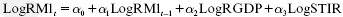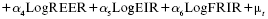(2)

where,

RM1(M1/CPI) 5 real narrow money balances

RM1t–1(M1/CPI)t–1 5 lagged value of real narrow money demand

RGDP 5 real gross domestic product

STIR 5 short term interest rate

REER 5 real expected exchange rate

EIR 5 expected inflation rate

α0 is the intercept term and μt is white noise error term.

To empirically analyze the long run relationships and dynamic interactions among narrow money and the regressors chosen, the following procedure was adopted. First, the properties of the times series used in the study were investigated in order to determine their order of integration.

The augmented Dickey–Fuller (ADF) and Philips–Perron (pp) unit roots tests were considered to be appropriate in the study. Second, the existence of a cointegraton relationship was investigated following the approach developed by Pesaran et al. , a procedure that has some econometric advantages over traditional approaches such as those of Engle and Granger , Johansen  and Johansen and Juselius . The advantages are three-fold: One, the procedure is applicable irrespective of whether the underlying regressors are purely I(0), purely I(1) or mutually cointegrated. Two, its statistical properties in small samples are superior to other tests of cointegration such that the latter are not robust when subjected to small sample sizes. Three, the use of the ARDL procedures ensures the estimation of the long and short run parameters of a model.

In the present investigation, two steps were followed: First, the Wald (F-test) was conducted to determine the existence of any long run relationship between narrow money and the chosen explanatory variables. Second, the long run relationship was estimated, followed by the short run coefficients using the error correction representation of the ARDL specification, with a view to establishing the speed of adjustment to equilibrium.

The ARDL model of the specification in Equation (2) above is: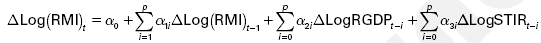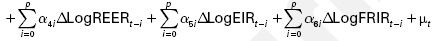(3)

Accordingly, the unrestricted error correction model (ECM) which follows the order of ARDL specification of narrow money demand of the above ARDL model is presented in Equation (4):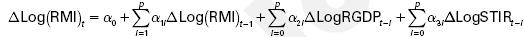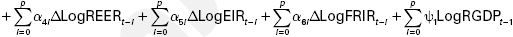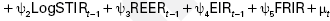(4)

where, the parameters αi : i 5 1, 2, 3, 4, 5 and 6 are the short run dynamic coefficients and Ψi: i 5 1, 2, 3, 4 and 5 are used to capture the long run multipliers of the underlying ARDL model.

A priori, the demand for money is expected to be positively correlated with real income, as there is a positive income effect on real cash balances. However, the magnitude of the income coefficient reflects the theoretical underpinning. Thus, the income coefficient is equal to unity, it is supportive to the quantity theory; if less than unity, the Baumol–Tobin inventory–theoretic approach applies; if it is greater than unity, the implication is that money can be considered a luxury. Real money demand is expected to be negatively correlated to interest rates (short or long term) because of the higher opportunity cost of holding money for example, when the interest rate becomes higher. In other words, if the interest rate becomes higher, ceteris paribus, less of money would be held and vice versa.

The elasticity coefficient of expected exchange rate variable can be either positive or negative, depending on how it is perceived by economic agents. Consequently, the coefficient is positive if depreciation (i.e., an increase in exchange rate) is perceived as an increase in wealth, whereby there is a rise in the demand for domestic money. On the other hand, there may be currency substitution, arising from decrease in exchange rate (i.e., appreciation of the domestic currency), whereby there is a corresponding decrease in the demand for domestic money, in which case the coefficient of exchange rate is negative. FRIR is expected to have negative relationship with the demand for money, in that depreciation of domestic currency and a rise in the FRIR may give rise to greater propensity to shift demand away from local currency to foreign currency, in line with the hypotheses of currency substitution and capital mobility. This is consistent with the view that when there is an increase in the FRIR, leading to higher returns on foreign assets relative to returns on domestic assets, there is the tendency that economic agents may reduce their holdings and thus the demand for domestic money.

Real money demand is inversely related to expected inflation rate, as expected rise in inflation increases the cost of holding money. Consequently, expected inflation has a lot of impact on the return of financial assets, since it imposes greater risks in saving money. Hence, ceteris paribus if the inflation rate is anticipated to rise, the demand for real money tends to decrease and vice versa. In essence, the coefficient of anticipated inflation rate is expected to have a negative sign, implying that the demand for real cash balances will tend to decrease if there are expectations that the price level will rise. The aim of incorporating the expected inflation rate in the money demand function is to measure the return on holdings of goods which are an alternative to domestic currency. The goods in question include equity holdings (shares), investment in land, building (real estate) and the like.

The presumptive signs of the variables are stated as follows:

α2 < 0, α3 < 0, α4 < 0, α5 < 0 and α6 < 0

To test the long run relationship among the variables, the Wald test (F-statistic) is conducted by imposing restrictions on the estimated long run coefficients. The null and alternative hypotheses are stated as follows:

Ho = a1 = a2 = a3 = a4 = a5 = a6 = 0 against the alternative hypothesis,

Ho ≠ a1 ≠ a2 ≠ a3 ≠ a4 ≠ a5 ≠ a6 ≠ 0

#### Results and Discussion

The testing procedure of the ARDL bounds test is performed in three steps. First, OLS is applied to Equation (4) to test the existence of a cointegrating long run relationship based on the Wald test for the joint significance of the variables. The optimal lag length for estimating the long term coefficients equation is selected using the schwarz bayesian criterion (SBC).

To select the optimal lag length for the model, we fit an autoregressive model of order 2 (AR 2), generating the results of the akaike information criterion (AIC) and the SBC. However, the study utilizes the results of the SBC in the choice of optimal lag length for the estimated model. The results of the selection are presented in Table 1.

Variables AIC
lag length
SBC
lag length
Lag length selected
0 1 2 0 1 2 AIC SBC
Log(RM1) 0.880563 -0.15724* -0.43525 1.133895 0.141346 0.124981* 1 2
Log(RGDP) 1.787436 0.732495 0.704963* 1.625701 1.031083* 1.265190 2 1
Log(STIR) 0.647156 -0.06706 0.054462* 0.900488 0.231533* 0.614689 2 1
Log(REER) 1.105565 -0.57901* -0.76785 1.358897 -0.28043 -0.20762* 1 2
Log(EIR) 2.280833 1.113569* 1.294407 2.534165 1.412157* 1.854634 1 1
Log(FRIR) 2.15363 1.605289 1.190868* 2.406962 1.903877 1.751095* 2 2

Table 1: Lag selection results.

From Table 1, it can be seen that for the chosen variables and the results of the estimation, the ARDL employed in the investigation is of the order (211212), on the basis of the SBC result. The results of the ADF and the PP tests for the variables under investigation are presented in Table 2.

Log(RM1) 0.728669 0.541945 -0.167440 -0.429498 -4.725127* -4.728453* -4.859184* -4.859184* I(1)
Log(RGDP) 1.973993 2.332115 -0.466067 -0.516276 -5.304534* -5.304534* -5.838140* -5.822947* I(1)
Log(STIR) -1.925603 -1.859225 -1.532214 -1.423815 -6.625502* -6.623123* -5.788376* -6.764757* I(1)
Log(REER) -3.925526* -2.365975 -3.885859** -2.009376 -3.545450 -2.561615 -3.916500 -2.729001 I(0)
Log(EIR) -2.214251 -1.979019 -2.027644 -1.691335 -2.876376*** -1.979019 -2.986774 -4.571960* I(1)
Log(FRIR) -2.129553 -1.209926 -3.757777** -1.908095 -4.757266* -4.663988* -4.821340 -5.089323* I(1)

Table 2: Unit root tests results.

From Table 2, it can be seen that the order of integration of the variables under investigation are mixed, a condition that lends credence to the use of the ARDL bounds test, as the mixture of both I(0) and I(1) variables would not apply under the Johansen procedure. The results of the bounds cointegration test are reported in Table 3.

Computed Wald (F-statistic): 3.48
0.10 0.05
k 5 6 I(0) I(1) I(0) I(1)
F* 2.12 3.23 2.45 3.61

Table 3: Critical values for ARDL bounds test.

It should be noted that the lower bound critical values assume that the explanatory variables are integrated of order zero, or I(0), while the upper bound critical values assume that the explanatory variables are integrated of order one, or I(1). Hence, if the computed F-statistic is less than the lower bound value, the null hypothesis is not rejected. On the other hand, if the computed F-statistic is greater than the upper bound value, the null hypothesis is rejected. However, if the computed F-statistic falls between the lower and upper bound values, the results are inconclusive.

Results in Table 3 suggest that the application of the bounds F-test using ARDL modelling approach indicates the existence of a long run relationship between narrow money demand, real income, STIR, REER, EIR and FRIR. The results show that the null hypothesis of no long run relationship is rejected at the 10% significance level. The Wald test (F-statistic) of 3.48 is greater than the upper critical bound values of 3.23. Therefore, the null hypothesis of no cointegration cannot be accepted at the 10% significance level and hence, there is a cointegration relationship between narrow money, real income, STIR, REER, EIR and FRIR. However, at the 5% significance level, the computed F-statistic value falls between the lower and upper bounds of the critical values. This is an inconclusive case which is subjected to further verification from the ECM results.

The next stage, having found a long run relationship among the variables, is to apply the ARDL model to estimate the long run and short run parameters. The results are shown in Panels A and B in Table 4.

Panel A: Long run estimates using ARDL based on SBC
Dependent variable: ∆Log(RM1)
Regressor Coefficient Standard error T-Statistic(probability)
C 1.337903 1.259889 1.061921(0.2965)
Log(RM1) 0.676607 0.163061 4.149406(0.0002)
Log(RGDP) 0.220263 0.107572 2.047582(0.0492)
Log(STIR) -0.314507 0.154400 -2.036967(0.0503)
Log(REER) 0.096796 0.144824 0.668373(0.5088)
Log(EIR) -0.098076 0.076832 -1.276494(0.2113)
Log(FRIR) -0.025942 0.117021 -0.221682(0.8260)
Diagnostics
R2 5 0.67; Adj. R2 5 0.61; F-statistic 5 10.48235(0.000002); SER 5 0.305089;
BG[2(2)] 5 11.72349(0.0028); JB 5 1.125807(0.569553); ARCH[2(1)] 5 0.033201(0.8554);
RESET 5 2.283669(0.1412).
Panel B: Short run estimates using ARDL based on SBC
Dependent variable: ∆Log(RM1)
Variables Coefficient Standard error T-Statistic(probability)
C -0.030425 0.031501 -0.965832(0.3427)
∆Log(RM1(21)) 1.040170 0.197045 5.278855(0.0000)
∆Log(RM1(22)) 0.621404 0.199654 3.112408(0.0044)
∆Log(RGDP(21)) 0.137937 0.080269 1.718438(0.0972)
∆Log(REER1) 0.272125 0.162221 1.677493(0.1050)
∆Log(REER(21)) -0.339524 0.218009 -1.557386(0.1310)
∆Log(REER(22)) 0.344648 0.159454 2.161428(0.0397)
∆Log(EIR) -0.092734 0.067929 -1.365156(0.1835)
∆Log(FRIR(21)) 0.170269 0.070719 2.407697(0.0232)
ECM(21) -0.907443 0.193285 -4.694841(0.0001)
Diagnostics
R2 5 0.60; Adj. R2 5 0.47; F-statistic 5 4.567041(0.001001); SER 5 0.141206;
BG[2(2)] 5 3.643637(0.1617); ARCH[2(1)] 5 0.027881(0.8674); JB[2(2)] 5 0.473537(0.789174);
RESET 5 0.016911(0.8975).

Table 4: Long run and short run estimates.

Long run estimates

The results of long run estimated narrow money demand function are presented in Panel A of Table 4. All the coefficients are correctly signed. There is a positive and statistically significant relationship between real narrow money demand and real income during the period of study, as the income elasticity is statistically significant at the 1% level. It implies that increase in real income leads to increase in the demand for money, as predicted by economic theory. The real income coefficient is 0.22 which is less than unity and is consistent with the transactions and precautionary theories as well as the Baumol–Tobin, inventory–theoretic approach because for M1, it is dominated by current transaction and precautionary needs. Consequently, the estimated income elasticity is supportive of the hypothesis of economies of scale in the demand for money, in line with transactions and precautionary theories.

The STIR has the correct sign and is negatively related to real narrow money demand, indicating the higher opportunity cost of holding money. The long run coefficient of –0.314507 is statistically significant at the 5% level.

The REER has a positive relationship with the demand for narrow money and is not statistically significant as indicated by the p-value. The relationship is consistent with theory that predicts that depreciation of domestic currency (i.e., an increase in exchange rate) can be perceived as an increase in wealth, leading to a rise in the demand for domestic money. Thus, exchange rate depreciation is probably perceived as an increase in wealth in Nigeria, although it is not a statistically significant determinant of narrow money demand in the long run.

The EIR has a negative sign, and is consistent with a priori expectations. Studies have found that changes in price level do predict changes in the demand for money. The coefficient of –0.098076 shows that the demand for narrow money in Nigeria will decrease by about 10% when the current inflation rate rises by 1%. If the intention of incorporating the expected inflation rate in the money demand function is to measure the return on items such as equity holdings (shares), investment in land and real estate, which are an alternative to holding domestic currency, the result indicates that the higher the rate of expected inflation (i.e., higher returns on the alternative assets), ceteris paribus, the lower is the demand for narrow money in Nigeria. Hence, people would tend to switch to other money alternatives when inflation is anticipated, because they promise higher rates of returns. The FRIR is correctly signed and is not a statistically significant factor explaining the demand for narrow money in Nigeria. A rise in the foreign real interest rate may give rise to greater propensity to shift demand away from local currency to foreign currency, in line with the hypotheses of currency substitution and capital mobility. Consequently the result shows that currency substitution exists in Nigeria.

The F-statistic value of 10.48235 is indicative of the overall statistical significance of the model at the 1% level as shown by the p-value. Thus, all the explanatory variables used in the model simultaneously explain the variations in the demand for real narrow money in Nigeria. The diagnostic statistics for the long run estimates are satisfactory. The adjusted R2 is 0.61%, implying that 61% of variation in the demand for narrow money is explained by lagged values of RM1t–1, STIR, REER, EIR and FRIR. Consequently, the coefficient of determination which measures the goodness-of-fit of the estimated model shows that the model has high predictive capability. The JB statistic 1.125807 and its associated p-value of 0.569553 indicate that the null hypothesis of normally distributed error term cannot be rejected. The autoregressive conditional heteroskedasticity (ARCH) statistic shows absence of heteroscedasticity. The RESET statistic is not statistically significant, implying the rejection of the null hypothesis of model misspecification.

Short run estimates

Hendry’s General to Specific technique was used in developing the short run estimated lagged ECM, by regressing Δlog(RM1) on its lagged values, the current and lagged values of the explanatory variables, in addition to the one period lagged residuals from the cointegrating Equation (3). The parsimonious results were attained following the application of variable deletion tests.

For the error correction representation, the demand for real cash balances is influenced by its own past values. Thus, the lagged values of cash balances (up to the second lag) are statistically significant at the 1% significant level and correctly signed. For a lag of 2, a 1% increase in past cash balances leads to an increase in demand for real cash balance by 62%.

The coefficient of real income is statistically significant at the 10%, while satisfying the priori expectations. Income is thus a significant determinant of the demand for narrow money in Nigeria in both the long and short run. The real income coefficient of 0.13 is less than unity and is predicted by the Baumol–Tobin, inventory–theoretic approach. The STIR was found to be statistically insignificant and was deleted in the process of generating the parsimonious results. This can be contrasted with the long run in which the coefficient is both correctly signed and statistically significant. Thus in the short run, interest rate does not seem to explain the demand for real narrow money in Nigeria, but an result that probably indicates the underdeveloped nature of Nigeria’s money market, which is deficient in depth and flexibility.

The REER (at 2 lags) is statistically significant at the 5% level and positively signed. This can be contrasted with the long run estimates which show the coefficient as not statistically significant. The EIR is correctly signed as in the long run and is not a statistically significant variable at the 10% level. However it is particularly interesting to note that in both the long and short run, the demand for real narrow money in Nigeria is inversely related to expected inflation. The FRIR coefficient is not correctly signed in the short run estimates, though it is statistically significant at the 10% level. The implication is that in the short run, FRIR would not tend to induce capital flight in contrast to the long run.

The ECM is negative and highly statistically significant, further lending credence to the cointegration among the variables under investigation. The feedback coefficient is –0.91 and suggests that about 91% disequilibrium is corrected in the current year. Hence, when narrow money demand is above or below its equilibrium level, it adjusts by approximately 91% within the first year to ensure full convergence to its equilibrium level. The speed of adjustment is thus very high. Overall, the results indicate that in the short run, change in real income and REER have significant impact on the demand for narrow money in Nigeria.

The goodness-of-fit for the short run ARDL model is approximately 60% and the adjusted R2 is 47%. In addition, the model satisfies the diagnostic Breusch–Godfrey serial correlation LM test. The F-statistic indicates joint significance of coefficients. The JB statistic is 0.473537 while the probability of obtaining the value, on the basis of the normality assumption is 79% implying that we cannot reject the null hypothesis of normally distributed error term. The null hypothesis of heteroscedasticity is rejected at the 1% level of significance, as indicated by the non-statistically significant ARCH statistic. The estimated model also satisfies Ramsey’s regression equation specification error test (RESET) it is not statistically significant, implying that the estimated model is correctly specified.

Stability of narrow money demand

To determine the stability of the demand for narrow money in Nigeria, two tests were conducted, i.e., the Chow breakpoint test and the cumulative sum of recursive (CUSUM) and cumulative sum of squares of recursive residuals (CUSUMSQ) tests, the results of which do not reject the null hypothesis of stability. It can be concluded that the results are suggestive that the narrow money demand function for Nigeria is stable over the study period.

For the Chow test, a breakpoint (1985) was chosen. The reason for choosing this point is that the reforms in the financial sector were intensified in 1986 when the structural adjustment programme (SAP) was embarked upon, with its prongs of liberalization, privatization and deregulation, on a scale previously unknown in the country. The Chow F-statistic is 1.871035 and is not statistically significant as indicated by its p-value of 0.4075. It is concluded that the demand for narrow money (M1) in Nigeria has not undergone structural change over the period of 1970 to 2010.

For the second test, to correct the disadvantage of the Chow test which assumes knowledge of the exact breakpoint, the CUSUM and CUSUMSQ tests were applied to the residuals generated from Equation (4). The CUSUM and CUSUMSQ plots do not cross the 5% critical lines, implying that the stability of real narrow money demand function exists over the entire sample period. The CUSUM and CUSUMSQ tests are shown in Figures 1 and 2.

Figure 1: CUSUM test.
The straight lines represent critical bounds at 5% significance level.

Figure 2: CUSUMSQ test.
The straight lines represent critical bounds at 5% significance level.

It is instructive to note that the stability test results are in contrast to several investigations conducted for both developed and developing countries, the primary purpose of which were to justify the appropriateness of existing money targeting frameworks, using various specifications and approaches and reporting unstable money demand function, occasioned largely by structural changes in the economy .

#### Conclusion

The paper aimed at estimating a narrow money demand function for Nigeria for the period 1970 to 2010. The ARDL procedure proposed by Pesaran et al. was employed in the empirical analysis. It was demonstrated that there is a long run relationship between narrow money demand, real income, STIR, REER, EIR and FRIR in the period under investigation. The results of the estimation indicate that in both long and short run, real income is a significant variable explaining the demand for narrow money in Nigeria, while interest rate is significant in the long run, it is insignificant in the short run. From the coefficients, it is clear that real income tends to be the most significant factor determining the demand of narrow money in Nigeria. The results also demonstrate that in the short run, Nigeria is not immune from external shocks originating from capital flight as evidenced by the coefficients of real expected exchange rate and foreign real exchange rate respectively. In addition, there is evidence that the narrow money demand function for Nigeria is stable over the sample period.

#### Competing Interests

The authors declare that they do not have any competing interests.

#### Authors’ Contributions

Both authors contributed equally to this work.

#### References

1. Simmons R, 1992. An error-correction approach to demand for money in five African developing countries. Journal of Economic Studies, 19: 29–48.
2. Nwafor F, Nwakanma H, Nkansah P, Thompson F, 2007. The quantity theory of money in a developing economy: empirical evidence from Nigeria. African Economic and Business Review, 5: 1–9.
3. Fielding D, 1994. Money demand in four African countries. Journal of Economics Studies, 21(2): 3–37.
4. Nell KS, 2003. The stability of M3 money demand and monetary growth targets: the case of South Africa. Journal of Development Studies, 39(3): 151–180.
5. Owoye O, Onafowora OA, 2007. M2 targeting, money demand and real GDP growth in Nigeria: do rules apply? Journal of Business and Public Affairs, 1: 1–20.
6. Kallon K, 1992. An econometric analysis of money demand in Ghana. Journal of Developing Areas, 26: 475–488.
7. Akinlo AE, 2006. The stability of money demand in Nigeria: an autoregressive distributed lag approach. Journal of Policy Modeling, 28(4): 445–452.
8. Bahmani-Oskooee M, Gelan A, 2009. How stable is the demand for money in African countries. Journal of Economic Studies, 36(3): 216–235.
9. Ghartey EE, 1998. Monetary dynamics in Ghana: evidence from cointegration, error correction modeling and exogeneity. Journal of Development Economics, 57: 473–486.
10. Drama BGH, Yao S, 2010. The demand for money in Cote d’Ivoire: evidence from the cointegration test. International Journal of Economics and Finance, 3(1): 2.
11. Bahmani-Oskooee M, Rhee HJ, 1994. Long-run elasticities of the demand for money in Korea: evidence from cointegration analysis. International Economic Journal, 8(2): 83–93.
12. Arize A, 1989. An econometric investigation of money demand behavior in four Asian developing countries. International Economic Journal, 3(4): 79–93.
13. Halicioglu F, Ugur M, 2005. On stability of demand for money demand function: evidence from a Developing OECD Country. Global Business and Economic Review, 7(3): 203–213.
14. Agénor PR, Khan M, 1996. Foreign currency deposits and the demand for money in developing countries. Journal of Development Economics, 50(1): 101–118.
15. Valdkhani A, Alauddin M, 2003. Demand for M2 in developing countries: an empirical panel investigation. Discussion Paper No. 149, Queensland University of Technology, Australia.
16. McCallum BT, 2001. Should monetary policy respond strongly to output gaps? American Economic Review, 91(2): 258–262.
17. Cziraky D, Gillman M, 2006. Money demand in an EU accession country: a VECM study of Croatia. Bulletin of Economic Research, 58(2): 73–159.
18. Mishkin FS, 2007. The Economics of Money, Banking and Financial Markets. Boston: Pearson–Addison Wesley.
19. Siklos PL, Barton AG, 2001. Monetary aggregates as indicators of economic activity in Canada: empirical evidence. Canadian Journal of Economics, 34: 1–17.
20. Laidler D, 1999. The Quantity of Money and Monetary Policy. Working Paper Series No. 99–5. Ottawa: Bank of Canada.
21. Hossain A, 1994. The Search for a Stable Money Demand Function for Pakistan: An application of the method Co-integration. Pakistan Development Review, 33: 969-981.
22. Ericsson N, 1998. Empirical modeling of money demand. Empirical Economics, 23(3): 295–315.
23. Brissimis SN, Hondroyiannis G, Swamy PAVB, Tavlas GS, 2003. Empirical modelling of money demand in periods of structural change: the case of Greece. Oxford Bulletin of Economics and Statistics, 65(5): 605–628.
24. Goldfeld SM, Sichel DE, 1990. The Demand for Money. In: Friedman BM, Hahn FH (Eds.), Handbook of Monetary Economics, Volume I. New York: Elsevier Science Publishers, 299–356.
25. Pesaran MH, Shin Y, Smith RJ, 2001. Bound testing approaches to the analysis of long run relationships. Working Paper, University of Cambridge.
26. Engle RF, Granger CWJ, 1987. Cointegration and error-correction: representation, estimation and testing. Econometrica, 55(2): 251–276.
27. Johansen S, 1988. Statistical analysis of co-integrating vectors, Journal of Economic Dynamics and Control, 12(2–3): 231–254.
28. Johansen S, Juselius K, 1990. Maximum likelihood estimation and inference on co-integration with applications to the demand for money. Oxford Bulletin of Economics and Statistics, 52(2): 169–210.
29. Lee TH, Chung KJ, 1995. Further results on the long run demand for money in Korea: a cointegration analysis. International Economic Journal, 9(3): 103–113.
30. Calomiris CW, Domowitz I, 1989. Asset substitution, money demand and the inflation process in Brazil. Journal of Money, Credit and Banking, 21: 78–89.
Select your language of interest to view the total content in your interested language

### Article Usage

• Total views: 12053
• [From(publication date):
May-2013 - Dec 06, 2019]
• Breakdown by view type
• HTML page views : 8230Can't read the image? click here to refresh